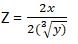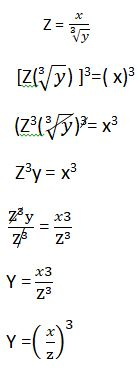KUULCHAT
S.H.S MATHEMATICS MOCK

#### OBJECTIVE TEST

1.

Bala sold an article for N6,900.00 and made a profit of 15%. calculate his percentage profit if he had sold it for N6,600.00.

A.
13%
B.
12%
C.
10%
D.
5%

selling price = N6,900.00

Profit percent = 15%

percentage profit if he had sold if for N6,600.00

let C.P represent cost price

S.P represnt selling Price

% represent Profit percent

C.P = S.P - P

but put P = %P X C.P

put P = 15% x C.P

P = 0.15 C.P

Gives = C.P = S.P - ( 0.15 - C.P )

but S.P = 6,900.00

C.P = 6,900 - (0.15 C.P )

Group like terms

C.P + 0.15 C.P = 6,900.00

1.5 C.P⁄1.5 = 6,900.00⁄1.5

therefore cost price of the article is 6,000

If the owner of the article had sold it N6,600.000

Profit = Selling price - Cost Price

= 6,600 - 6000

N600.00

since profit is know

percent profit = P⁄C.P x 100

= 600⁄6000 x 100

10%

2.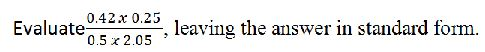A.

1.639 X 10-2

B.

1.639 X 10-1

C.

1.639 X 101

D.

1.639 X 102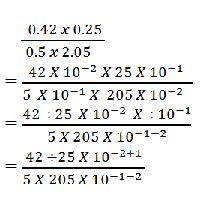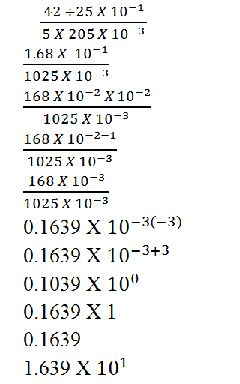3.

If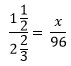, find the value of x.
A.

42

B.

45

C.

54

D.

64

Change the mixed fractions to improper fractions

Rule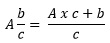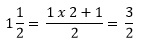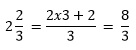Rules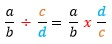Thus, reciprocate/switch the numerator and denominator at the right and change the division to multiplication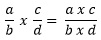Thus, the numerators multiply each other and the denominators multiply each other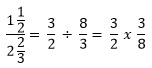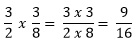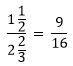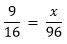Multiply both sides by 96

96 x 9/16 = x

16 goes into 96, 6 times

6 x 9 = x

x = 54

4.

The total surface area of a solid cylinder is 165cm2.

If the base diameter is 7cm, calculate its height [Take ϖ = 22⁄7]

A.
2.0 cm
B.
4.0 cm
C.
4.5 cm
D.
7.5 cm

diameter = 7cm

Area of cylinder = 165cm2 ϖ = 22⁄7

165 = 8(22⁄7)(3.5)(h) + 2(22⁄7)(3.5)2

165 = 22h + 44⁄7 (12.25)

165 =22h + 77

f=group like terms

165 - 77 = 22h

88 =22h

divide through by 22

h=4

5.

solve 4x2 - 16x + 15 = 0

A.

A. x = -1 1⁄2 or -2 1⁄2

B.

B. x = 1 1⁄2 or -2 1⁄2

C.

C. x = 1 1⁄2 or 2 1⁄2

D.

D. x = 1 1⁄2 or -2 1⁄2

multiply the co-efficient of x 2 which is 4 with 5 = 60

look for two numbers If you add it will give you the co-efficient of x and multipy will give 60

The two numbers are -10,-6

substitute -10,-6 in place of the co-efficient of x which is -16

Gives 4x2 - 10x - 6x + 15 = 0

Group like terms

(4x2 - 6x ) - (10x + 15)

2x(2x - 3) - 5(2x - 3) = 0

(2x -5)(2x -3) = 0

2x -5 = 0

2x⁄2 = 5⁄2

x = 5⁄2 = 2 1⁄2

2x - 3 = 0

2x⁄2 = 3⁄2

x = 3⁄2 = 1 1⁄2

x = 1 1⁄2 or 2 1⁄2

6.

3p = 4q and 9p = 8q - 12, find the value of pq

A.
-12
B.
-7
C.
7
D.
12

3p = 4q ...... 1eqn

9p = 8p - 12 .......2eqn

multiply equation 1 by 3

3 x 3p = 3 x 4q

9p = 12q ..... 3eqn

put 3eqn into 2eqn

12q = 8q - 12

12q - 8q = -12

4q⁄4 =-12⁄4

q = 3

put q = -3 into 1

3p = 4(-3)

3P⁄3 = -12⁄3

Pq = (-4)(-3)

12

7.

Two friends, Dede and Kofi decided, to buy the same type of car. They found out that the car cost ₦3,000,000.00. The amount of money (f) which Dede had was not enough to buy the car but Kofi had enough money (k) to buy the car. Which of the of the following inequalities is true?

A.

f≤₦3,000,000.00 ≤ k

B.

f> ₦3,000,000.00 > k

C.

f< ₦3,000,000.00 ≤ k

D.

f≥ ₦3,000,000.00 ≥ k

The amount of money(f) which Dede has was not enough to buy the car
⇒ f(Dede's amount) < (less than) the cost of car (₦3,000,000.00)
⇒ Kofi can have the exact amount of the car or even more than the cost of the car
⇒k≥₦3,000,000.00
⇒ f< ₦3,000,000.00 ≤ k
Read as Dede's amount(f) is less than ₦3,000,000.00 and ₦3,000,000.00 is less than or equal to Kofi's amount(k)

8.

If log102 = 0.3010 and log102y = 1.8060, find, correct to the nearest whole number, the value of y.

A.

7

B.

6

C.

5

D.

4

Applying logabc = c x logab = clogab

log102y = y x log102

But log102y = 1.8060

⇒ y x log102 = 1.8060

But log102 = 0.3010

Hence y x 0.3010 = 1.8060

Dividing both sides by 0.3010

⇒ y = 1.8060/0.3010 = 6

9.

A man bought a car which costs ₦5,000,000.00 from a dealer on hire purchase. He pays a deposit of ₦3,000,000.00 and agrees to pay the balance at 8% compound interest per annum. If he pays ₦1,000,000.00 at the end of each year, how much will be remaining to be paid after two years?
A.
₦92,800.00
B.
₦252,800.00
C.
₦320,000.00
D.
₦332,000.00

Cost of car = ₦5,000,000.00

Deposite = ₦3,000.000.00

Rate = 8% compound intesrest per annum = 8100 = 0.08

Balance = ₦5,000,000.00 - ₦3,000,000.00 = ₦2,000,000.00

Hence the interest will only be charged on the ₦2,000,000.00 balance after the first year

Fisrt year

Interest = Principal x Rate x Time

Time = 1 (Compound interest is calculated year by year)

Interest = 0.08 x ₦2,000,000 = ₦160,000

Total amount to be paid by the end of the first year
⇒ ₦2,000,0000 + ₦160,000 = ₦2,160,000

Since he pays ₦1000,000 at each year

Amount left to pay the subsequent year
⇒ ₦2,160,000 - ₦1,000,000 = ₦1,160,000

Second Year

Interest = 0.08 x ₦1,160,000 = ₦92,800

Amount to pay
⇒ ₦92,800 + ₦1,160,000 = ₦1,250,8000

He pays another ₦1,000,000 by the end of the second year.

Hence amount to pay after the second year
⇒ ₦1,252,800 - ₦1,000,000.00 = ₦252,800

NB: For compound interest, the new principal amount is the interest + the previous amount to be paid

10.

Find the equation whose roots are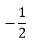and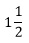.
A.

2x2 -4x + 6 = 0

B.

4x2 -4x - 3 = 0

C.

2x2 +3x + 4 = 0

D.

4x2 -4x + 3 = 0

Change the mixed fraction to improper fraction

Rule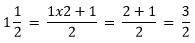If α and β are the two roots of a quadratic equation, then the formula to construct the quadratic equation is:

x2 - (α + β)x + αβ = 0

Thus, x2 - (sum of roots)x + product of roots = 0

Sinceandare the roots

x2 - (-1/2 + 3/2)x + (-1/2 x 3/2) = 0

-1/2 + 3/2 = (-1+3)/2 = 2/2 = 1

Rule-1/2 x 3/2 = (-1x3)/(2x2) = -3/4

x2 - (1)x + (-3/4) = 0

x2 - x - 3/4 = 0

NB: + - = - ⇒ + - 3/4 = -3/4

Multiply both sides by 4

4x - 4x - 3 = 0

11.

find the equation of a straight line passing through the point (1,-5) and having gradient of 3⁄4

A.

3x-4y-23 = 0

B.

3x-4y+23 =0

C.

3x+4+23 =0

D.

3x+4y-23= 0

Equation of a line y = mx + c

If point (1,-5) is given and gradient 3⁄4

y = -5

x = 1

y = mx + c

Gives : -5 = 3⁄4 (1) + c

-5 = 3⁄4 + c

Group like terms

-5-3⁄4 = c

-5⁄1 -3⁄4 = c

-20-3⁄4 = c

-23⁄4

y = 3⁄4x - 23⁄4

multipy through by 4

4 x y = 4 X 3⁄4x - 23⁄4 x 4

4y =3x - 23

0 =3x - 23 - 4y = 0

3x - 4y - 23 = 0

12.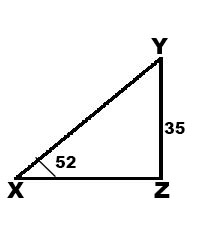Not drawn to scale

In ∆XYZ, ∣YZ∣ = 32 cm, ∠YXZ = 52 ° and ∠XZY = 90 °.

Find, the correct to nearest centimetre. ∣XZ∣.

A.
13cm
B.
20 cm
C.
25 cm
D.
31 cm∅ = 52

opposite ∣YZ∣ = 32

since opposite is known and we are ask to find adjacent (∣XZ∣)

which says tan∅ = opposite⁄Adjacement ∣YZ∣⁄∣XZ∣

tan(32) = 32⁄∣XZ∣

cross multiply

tan32⁄1 = 32⁄∣XZ∣

∣XZ⁄ tan(52) =32

On your scientific calculator tan(52) = 0.52

∣XZ (∅.28) = 32

making ∣XZ∣ the subject divide through by 0.53

∣XZ⁄0.53 = 32⁄1.28

∣XZ = 32⁄1.28

∣XZ∣ = 25cm

13.

If 6 and 14 are consective terms in arithmetic progression (A.P), find the value of p.
A.
8
B.
6
C.
10
D.
9

let represent initial term d represent difference

a = 6

d = common diffence

To find common diffence is the second term minus the first time that's current minus intial term

d = p - 6 = 14 -p

p - 6 = 14 - p

Group like terms

p + p = 14 + 6

2p⁄2 = 20⁄2

p = 10

14.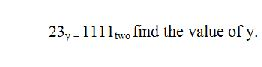A.
7
B.
6
C.
5
D.
4

1st expand the equation by multipyling

number y from zero, alway number it from your right to left do same to the other side of the equatiion

(2xy1) + (3 x y0) = (1x23)+ (1x22) + (1x21) + (1x20)

2y + 3 = 8 + 4 + 2 + 1

Grouping like terms

2y = 8 + 4 + 2 + 1

2y⁄2= 12⁄2

y = 6

15.

y varies inversely as the square of x. When x = 3, y = 100. Find the value of x when y = 25.

A.

x = 30

B.

x = 12

C.

x = 6

D.

x = 5

y1x2

NB: ∝ is the symbol for proportional
inversely proportional is 1 over the expression
directly proportional is just the expression (It would had been yx2)

a constant(=k) is introduced in place of the ∝
y = kx1x2
y = kx2

Use the known values to calculate the constant(k) to get the general expression

y = 100 and x = 3
⇒ 100 = k32
100 = k9
100x9 = k
k = 900
y = 900x2

when y = 25
⇒ 25 = 900x2
25 x x2 = 900
x2 = 90025
x2 = 36
x2 = √36
x = 6

NB: The square root of a squared number or variable is equal to the number or variable

16.

An empty rectangular tank is 250 cm long and 120 cm wide. If 180 litres of water is poured into the tank, calculate the height of the water.

A.

4.5 cm

B.

5.0 cm

C.

5.5 cm

D.

6.0 cm

Let h = height of the water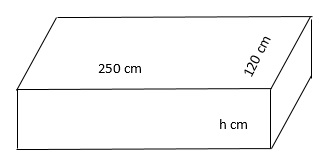Volume of a cuboid = Length x Breadth x Height

Since the length and breadth are in cm, convert the litres to cm3

1 litre = 1000 cm3

⇒ 180 litres = 180 x 1000 cm3

Length = 250 cm, breadth = 120 cm

Volume = 180 litres = 180 x 1000 cm3

⇒ 250 cm x 120 cm x h cm = 180 x 1000 cm3

Divide both sides by 250 cm x 120 cm

h = (180 x 1000) / (250 x 120) = 6

17.

Arrange the following in ascending order of magnitude: 0.45,¾ and 25%

A.

¾, 0.45, 25%

B.

¾, 25%, 0.45

C.

0.45, 25%, ¾

D.

25%, 0.45, ¾

Change the fraction and decimal into percentage so that they will all be in percentages.

Percentage is simply multiplying by 100.

0.45 ⇒ 0.45 x 100 = 45%

¾ ⇒ ¾ x 100 = 75%

Now arrange in ascending order

25%, 45%, 75%

45% = 0.45

¾ = 75%

Hence the ascending order is 25%,0.45,¾

NB: Ascending is from lowest to highest

18.

If (0.25)y find the value of y.

A.
-5⁄2
B.
-3⁄2
C.
3⁄2
D.
5⁄2

(25⁄100)y = 32

(1⁄4)y 32

log(1⁄4)y = log32

ylog 0.25 = log32

y = log32⁄log0.25

y = -2.5

y = -25⁄10

y = -5⁄2

19.

There are 8 boys and 4 girls in a lift.what is the probability that the first person who steps out of the lift will be a boy

A.

1⁄4

B.

2⁄3

C.

1⁄3

D.

3⁄4

Number of Boys = 5

Number of Girls = 4

Total pupils = 8 + 4

To get the probability of boys out of lift is the number of boys over the total number of pupils

P(a boys steps out of the lift) = 8⁄12

2⁄3

20.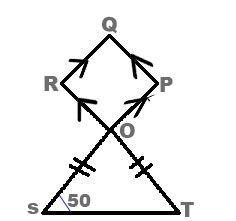In the diagram,POS and ROT are straight lines.QPQP is a parallelogram,

|QS| = |QT| and ∆OST = 50°; Calculate tyhe value of ∆OPQ

A.
160°
B.
140°
C.
120°
D.
100°

∆OST = OTS (Isocicoles)

OTS = 50°

considering ∆OST

50 + 50 +∆SOT = 180

100 + ∆SOT =180

Group like terms

∆SOT = 180

∆SOT = 80°

∆SOT = ∆ROP = 80° (vertical opposite angles are equal

80 + OPQ = 180

OPQ =180 - 80

OPQ = 100

21.

Given that 3 x 91 + x=27-x,find the value of x.
A.
-35
B.
-123
C.
-2
D.
-3

3 x 9 1 + x = 27-x

Concept

Ab x Ac = Ab+c

Thus if the bases are the same, we can add the indexes (superscripts)

3 can go into the other numbers(9,27) hence we change then to have base 3

9 = 31x 31 = 31 + 1=32

27 = 31x 31 x 31 =31+1+1 = 33

⇒ 3 x 32(1 + x) = 33(-x)

But 3 = 31, thus every number is raised to the power 1
⇒ 31 x 32(1 + x) = 33(-x)

Applying Ab x Ac = Ab + c

3 1 x 32(1+ x) = 31 + 2(1 + x )

31+2(1+ x) = 33(-x)

Concept
If Ab = Ac ⇒ b = c

Thus if the base at the left side of the equation, is the same as the right side of the equation, then the powers are the same

∴ 1 + 2(1 + x ) = 3(-x)

Concept

a(b + c ) = a x b + a x c

a(b - c ) = a x b - a x c

Thus the number outside the bracket multiplies each of the number in the bracket and the sign ( + or - ) is(are) maintained

-x = -1 x x

3(-x) = 3 x (-1) x x = -3x

1 + 2(1 + x ) = 1 + 2 x 1 + 2 x x

= 1 + 2 + 2x

= 3 + 2x

1 + 2(1+x) = 3(-x)

⇒ 3 + 2x = -3x

Grouping the x at the left and the rest at the right

Concept
If the sign is positive(+) and crosses to the other side of the equation the sign changes to negative ( - ) and the vice versa

2x + 3x = -3

5x = -3

Concept
Divide both sides by the number multiplying the number or variable (x)

5x5 = -35

x = -3⁄5

22.

In a class of 39 student, 25 offer Fante and 19 offer Twi. Five student do not offer any of the two languages. How many student offer only Twi?
A.
6
B.
7
C.
8
D.
9

Use a venn diagram to illustrate

In a class of 39 student ⇒ n(u)=39
let F represent students who offer Fante ⇒ n(F)= 25
Let T represent students who offer Twi ⇒ n(T)= 19
Five student do not offer any of the two languages ⇒ 5 are outside the sets F and T
Let n represent number of student who offer both languages

NB: You need to substract the number of the students who offer both languaes from each of the languages to get the students who offer only that languages.

When we add all the parts in the venn diagram we are to get the total number of students n(u) = 39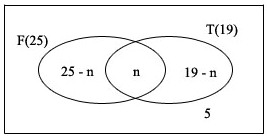(25 - n )+ n + (19 - n) + 5 =39
25 - n + n + 19 -n + 5 = 39
-n + 49 = 39
-n = 39 - 49
-n = -10
-n-1 = -10-1
n = 10
Only Twi = 19-n
⇒ Only Twi = 19 - 10 = 9

23.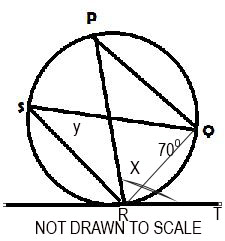In the diagram, RT is a tangent to the circle at R,∠PQR =70° , ∠QRT =52°re; ∠QSR = y and ∠PRQ = x. Use the diagram to answer question 40 and 41

A.
18°
B.
52&°
C.
60°
D.
45°∠TRQ = ∠RSQ = 52°

y = ∠RPQ = 52

24.

Express, correct to three significant figures, 0.003597.
A.
0.00359
B.
0.00360
C.
0.004
D.
0.359

Starting zeros are not significant and so the number of significant digits starts from 3597. The 3 digits lands on 9 but the digit next to it is more than 5 (7) and so 1 is added to the 9 to make it 10 and so the 0 is written down and the 1 is added to the 5 next to it which makes it 6.

NOTE
Zeros between other digits are significant. Only starting zeros are insignificant. For instance 3.052306, all the zeros are significant because they are not starting zeros after a decimal point.

25.

If T = {prime numbers} and M = {odd number} are subset of U = {x : 0 < x ≤}, and x is an interger,

find (T1 n M 1)

A.
{1,2,3,5,7,8,9}
B.
{1,2,4,6,8,10}
C.
{1,4,6,8,10}
D.
{4,6,8,10}

T ={ 2,3,5,7}

M ={1,3,5,7,9}

U {1,2,3,4,5,6,7,8,9,10}

T1 is called T compliment, which means numbers found in universal set but can't be found in set T

M1 is called M compliment, which means numbers found in universal set but can't be found in set M

T1 = {1,4,6,8,9,10}

M 1 = { 2,4,6,8,10}

T 1 M 1 = { 4,6,8,10}

26.

Find the angle which arc of the length 22 cm subtends at the centre of a circle of radius 15cm. [Take π = 22⁄7 ]

A.
156°
B.
96°
C.
84°
D.
70°

Length of an arc = 22 cm

π = 22⁄7

angle (∅)= ?

formular for length of an arc = ∅⁄360 x 2πr

since length of an arc =22 cm

r = 15 and π = 22⁄7

22 = π⁄360 X 2(22)⁄7 X 15

22 = π⁄360 X 44⁄7

22 = π⁄360 X 94.286

22 = 94.2860⁄360

22⁄0.262 = 0.2620⁄0.262

π =22⁄0.262

π = 84

The angle is 84 °

27.

(27.63)2 - (12.37)2

A.
610
B.
611
C.
612
D.
614

diffence of two squards

(27.63 - 12.37)(27.63 + 12.37)

610.4

Run it to three dicimal place

since 4 is less than 5 you maintian it

28.

Eric sold his house through an agent who charged 8% comission on the selling price.If Eric recieved \$117,760.00 after the sale, what was the selling price of the house ?
A.
\$120,000.00
B.
\$125,000.00
C.
\$128,000.00
D.
\$130,000.00

Commision on selling price let selling price of house = x

commision on selling price = x x 8⁄100

\$0.08x

Eric share of the house = 17,760.00

Total selling price of the house = Agent commision + Eric share x = \$117,760 + \$0.80x

group like terms

x - 0.08x = 117,760.00

0.92x⁄0.92 = 117760⁄0.92

x = \$178,000.000

The total selling price of the house is 128,000

29.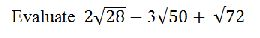A.
4√7 + √2
B.
4√7 - 9√2
C.
4√7 - 11√2
D.
4√7 - 21√2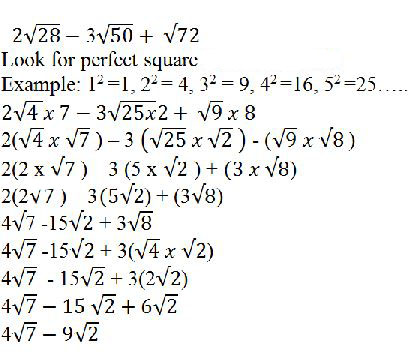30.

If 2a = √64 and b⁄a = 3, evaluate a2 + b2

A.
48
B.
90
C.
160
D.
250

Considering 2a = √64

2a = √64

what raise the power 2 will give you 64

26 = √64

2a = 261⁄2

since √ = 1⁄2

2a = 26(1⁄2)

divide 2 by 6

2a = 23(1)

2a = 22

since the base arethe same we equate the exponent considering b⁄a = 3

b⁄a = 3

cross multiple

b⁄a = 3⁄l

b(1) = 3(a)

b =3a

since a = 3

b = 3(3)

b = 9

therefore a2 + b2 = 92 + 32

81 + 9

90

31.

Simplify: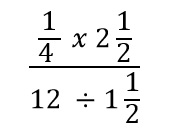A.
5⁄8
B.
5⁄32
C.
5⁄64
D.
5⁄72

C

NB: Change the mixed fractions to improper fractions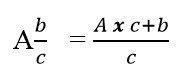Explanation:

Multiply the whole number(A) by the denominator(c) and add the numerator(b) to the result(Axc). Divide everything by the denominator(c).

⇒ 212 = (2x2 + 1)2 = 52
⇒ 112 = (1x2 + 1)2 = 32

Concepts

a = a/1
b = b/1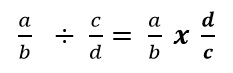Explanation:

Change the ÷ to x (multiplication). To do so:
Reciprocate the fraction at the right side of the ÷
Reciprocate means the top goes to the down and the down comes to the top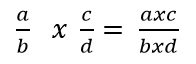Explanation:

The numerators multiply each other over the denominators multiplying each other.

Thus the products of the numerators over the products of the denominators

Product means multiplication

Applying all the above concepts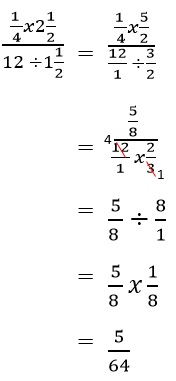32.

If the sequence x,4,16,y is Geometric Progression (GP), find the ratio of x:y.

A.

64:1

B.

8:1

C.

1:3

D.

1:64

Geometric sequence is a sequence of numbers where each term after the first is found by multiplying the previous one by a fixed, none-one number called the common ratio

Rules

For the sequence a1, a2, a3, a4 ...

Where a1 = first term

Where a2 = second term

Where a3 = third term

Where a4 = forth term

r = a2 / a1 = a3 / a2 = a4 / a3

Where r = common ratio

Thus the common ratio (r) is the same for all calculations

Hence 4/x = 16/4

4/x = 4

Multiplying both sides by x

x x 4/ x = 4 x x

4x = 4

Dividing both sides by 4

x = 1

16/4 = y/16

4 = y/16

Multiplying both sides by 16

16 x 4 = y/16 x 16

y = 64

⇒ x:y = 1:64

33.

A ladder, 10 m long, touches a side of a building at a height of 8 m. At what height would a ladder with length 12 m touch the building, if it makes the same angle with the ground?

(Assume that the ladder and building are on the same horizontal ground)

A.

10.6 m

B.

10.4 m

C.

10.0 m

D.

9.6 m

Represent them by diagrams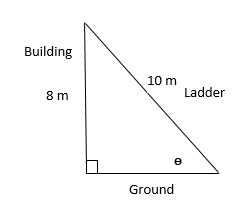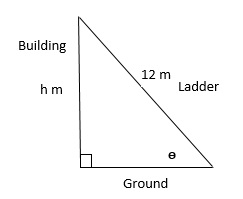The angle θ can be calculated using any of SOH, CAH , TOA

SOH = sin θ = Opposite (O) / Hypotenuse (H)

CAH = cos θ = Adjacent (A) / Hypotenuse (H)

TOA = tan θ = Opposite (O) / Adjacent (A)

You have to know which of the sides are known (Adjacent, Opposite or Hypotenuse)

The hypotenuse is the side facing the right angle triangle (90o), the ladder

The opposite is the side opposite (facing) the angle θ, the building

From both diagrams, the Opposite and Hypotenuse sides are known

Hence SOH can be used to calculate the angle

SOH = sin θ = Opposite / Hypotenuse

Opposite = 8 m and Hypotenuse = 10 m

Hence sin θ = 8 m / 10 m = 8 / 10

Opposite = h, Hypotenuse = 12 m

Hence sin θ = h m/ 12 m

Since the angle (θ) is the same as indicated in the question, it means the value of sin θ is the same for both the first and second ladders

Hence 8/10 = h/12

Multiply both sides by 12

12 x 8/10 = h

∴ h = 9.6

34.

Calculate the value x.

A.
48°
B.
55°
C.
58°
D.
70°

Considering ‣RPQ

Sum of interior angle is 180°

∠PRT + ∠RQP + ∠RPQ = 180

x+70+52 = 180

x+122 = 180

x =180 - 122

x=58°

35.

Find the truth set of the equation (x -2)2 + 3 = (x + 1)2 - 6.

A.

{-2}

B.

{-1}

C.

{1}

D.

{2}

Concept

(a+b)2 = a2+2xaxb + b2 = a2+2ab + b2
(a-b)2 = a2-2xaxb + b2 = a2-2ab + b2

Alternatively
(a+b)2 = (a+b)x(a+b) [Expand and simplify]
(a-b)2 = (a-b)x(a-b) [Expand and simplify]

⇒ (x-2)2 = x2-2xxx2+22 = x2-4x+4
(x+1)2 = x2+2xxx1+12 = x2+2x+1

(x-2)2+3 = (x+1)2 - 6
x2-4x+4+3 = x2+2x+1 - 6
x2-4x+7 = x2+2x-5
x2-x2-4x-2x = -5 - 7
-6x = -12
-6x-6 = -12-6
x = 2

36.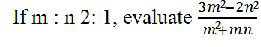A.
3⁄5
B.
3⁄4
C.
5⁄3
D.
4⁄3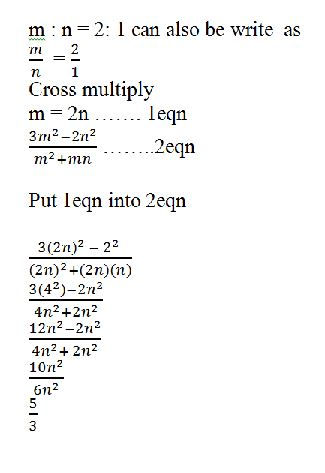37.

Simplify: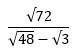A.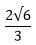B.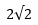C.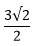D.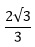3 can go into 48, hence express 48 as a multiplication of 3 and 16

√48 = √(16x3)

Applying √(axb) = √a x √b

⇒ √(16x3) = √16 x √3

√16 = 4

Hence √16 x √3 = 4 x √3 = 4√3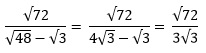NB: 4√3 - √3 is similar to 4x - 3x, thus you can simplify like terms.

Express 72 too as a multiplication of 24 and 3 so we can cancel the √3 at the numerator and denominator

√72 = √(24 x 3) = √24 x √3

24 = 4 x 6

√24 = √(4 x 6) = √4 x √6

√4 = 2

√24 = 2 x √6 = 2√6

⇒ √72 = √(24 x 3) = √24 x √3 = 2√6 x √3

⇒ √72 /3√3 = 2√6 x √3 / 3√3

√3 cancels each other

Hence 2√6/3

38.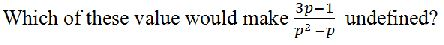A.
-1
B.
1⁄3
C.
- 1⁄3
D.
1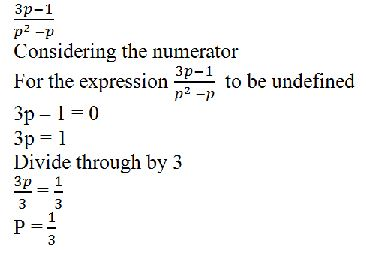39.

If y varies inversely as x and y = 6 when x = 3, find y when x = 9

A.

4

B.

3

C.

2

D.

1

y ∝ 1 / x

NB: Inversely = 1 / the relation

Varies directly = Relation 1 ∝ Relation 2

Find the constant of the relation

Let k = the constant

y = k x 1/x

When y = 6, x is 3

⇒ 6 = k/3

Multiplying both sides by 3

⇒ k = 6 x 3 = 18

Hence y = 18/x

When x = 9

⇒ y = 18 / 9 = 2

40.

The graph is for the relation: y = d - x.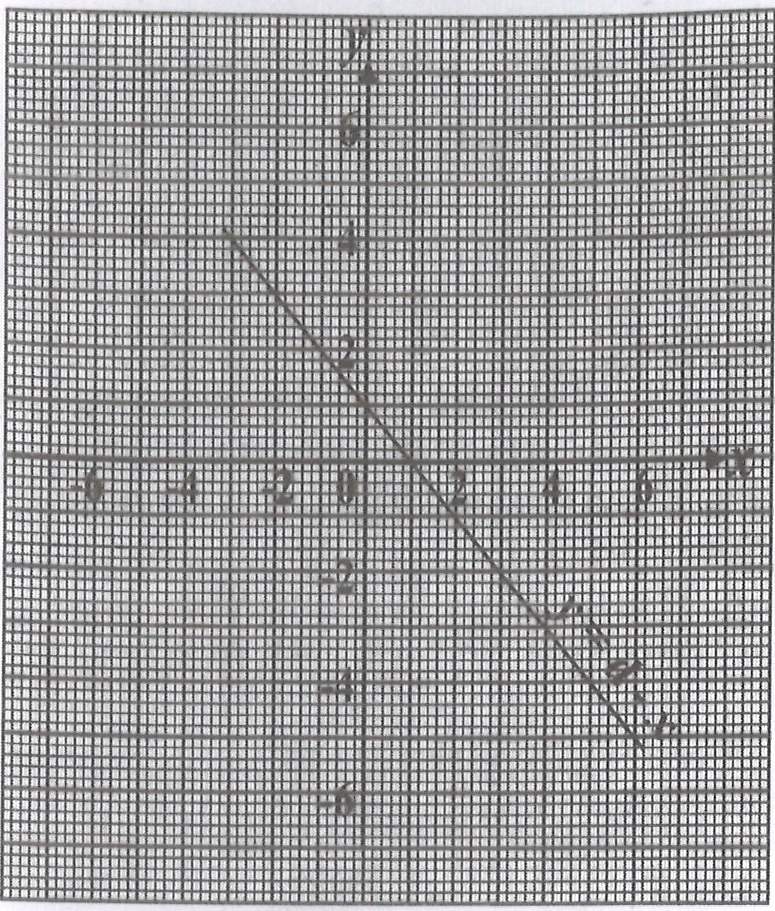Find the value of d.

A.

2

B.

1

C.

0

D.

-1

Trace any point on the graph where the line passes and use that x and y coordinates to substitute and find y

The line passes through the points (0,1), hence x = 0 and y = 1

y = d - x ⇒ 1 = d - 0 ⇒ 1 = d ⇒ d = 1

NB: The line also passes through the points (1,0), (4, -3 ). Any point on the line you use should arrive at the same answer

41.

The interior angle of a polygon are 3x°;2x°;4x°; 3x°; and 6x°. Find the size of the smallest angle of the polygon.

A.
30°
B.
40°
C.
60°
D.
80°

Since the angle of the polygon are five

It means the polygon is pentagon

interior angles of polygon = n-2x180⁄n

number of side (n) = 5

Interior angles of pentagon = (5-2)X180⁄5

=3X180⁄5

=540⁄5

108

since 180 is the interior

3x which is the smalllest angle

3x⁄3 = 108⁄3

x =60°

the smallest angle is 60°

42.

Solve the equation: t - 95 = -1115 .

A.

t = 3⁄5

B.

t = 11⁄15

C.

t = 4⁄5

D.

t = 13⁄15

Change the mixed fraction to an improper fraction.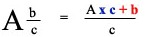Explanation:
Multiply the whole number(A) by the denominator(c) and add the numerator(b). Divide everything by the denominator(c).

⇒ 1115 = (1x15+1)15 = 1615

⇒ t - 95 = -1615

Get ride of the denominators by multiplying both sides by the least common multiple(LCM)
The denominators are 5 and 15,5 can go into 15, hence the LCM is 15

tx15 -15x95 = 15x-1615
15t - 27 = -16
15t = -16+27
15t = 11
15t15 = 1115
t = 1115

43.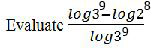A.
-1⁄2
B.
1⁄3
C.
1⁄2
D.
-1⁄3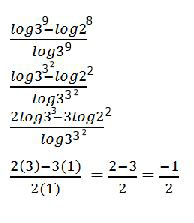44.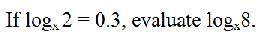A.
0.6
B.
0.9
C.
1.2
D.
2.4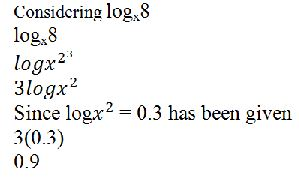45.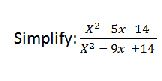A.

x+2⁄x-2

B.

x-2⁄x+4

C.

x+7⁄x-7

D.

x-7⁄x-7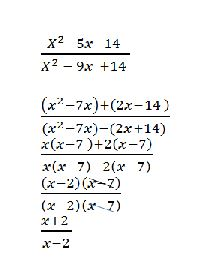46.

A rectangular board has length 15 cm and width xcm.If the side are doubled,find its new area

A.

15x cm2

B.

30x cm2

C.

45x cm2

D.

60x cm2

Length = 15cm

width = x cm

If the side are double

New length = 15 X 2

30cm

New width = 2 X x

2x

2x

Area of new rectangular box = Length(L) X (W)

30cm X 2xcm

60x cm2

47.

Make x the subject of the relation: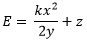A.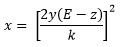B.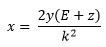C.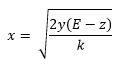D.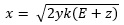Move the z to the left side, + becomes - and - becomes +

E - z = kx2/2y

Multiply both sides by 2y to get rid of the 2y at the denominator

2y x (E-z) = kx2

2y(E-z) = kx2

Divide both sides by k

2y(E-z)/k = x2

To get rid of a square on an alphabet, take the square root of both sides

Thus48.

Evaluate (0.064)-1/3.
A.
-5/2
B.
-2/5
C.
2/5
D.
5/2

Change the decimal number to a standard form with a complete whole number
∴ 0.064 = 64 x 10-3
⇒ (0.064)-1/3 = (64 x 10-3)-1/3
Applying, (a x b)n = an x bn ⇒ (64 x 10-3)-1/3 = 64-1/3 x 10(-3 x -1/3)
64-1/3 x 10(-3 x -1/3) = 64-1/3 x 101
Note:-3 x -1/3, negatives cancels each other likewise the 3s
Change the 64 to an indices form in order to cancel the powers
64 = 2 x 2 x 2 x 2 x 2 x 2 = 26
⇒ 64-1/3 x 101 = (26)-1/3 x 10
3 goes into 6, 2 times ⇒ 6 x -1/3 = -2
∴ (26)-1/3 x 10 = 2-2 x 10
Applying, a-n = 1 / an
⇒ 2-2 = 1 /22
22 = 4
∴ 2-2 x 10 = 1/4 x 10
Using 2 to cancel each other, 2 goes into 4, 2 times and 10, 5 times
⇒ 1/4 x 10 = 1/2 x 5 = 5/2
Note 1/2 x 5 = 1/2 x 5/1 , every number is divided by 1
Applying a/b x c/d = axc / bxd
⇒ 1/2 x 5/1 = 1 x 5 / 2 x 1 = 5/2
∴ (0.064)-1/3 = 5/2

49.

Factorize completely

(2x2y)(x-y) + (2x -2y)(x + Y).

A.

2(x - y)

B.

2(x - y)(x + Y)

C.

4(x-y

D.

4(x - y)(x + y)

Expand the bracket

2x(x) +2y(x-y) +2x(x+y) 2y(x+y)

2x2 - 2xy + 2xy + - 2y2 + 2x2 + 2xy - 2xy - 2y2

2x2 - 2y2 + 2x2 + 2y2

Group like terms

2x2 - 2y2 - 2y2

factorize 4 out

4(x - y2)

(x - y2) = (x - y)(x + y)

4[(x - y)(x + y)]

50.

In the diagram O is the centre of the circle with raduis 18 cm. If the angle ∠ZXY = 70° , calculate the length of arc ZY. [Take π = 22⁄7

A.
80 cm
B.
44 cm
C.
22 cm
D.
11 cm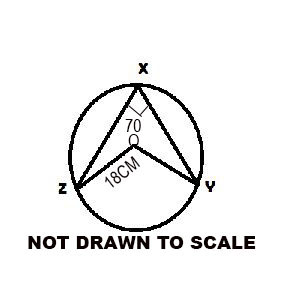∠ZXY = ∠ZOY = 70°

Length of an arc = Δ⁄2πr

since angle (Δ)π = 22⁄7 and r = 18cm

length of an arc =70⁄360 x 2 x 22⁄7 x 18

cancelation will take place here

70⁄360 x 2 x 22⁄7 x 18

1⁄18 x 22 x 18

22cm

#### THEORY QUESTIONS

1.
If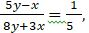find, correct to two decimal places, the value of x
manipulate the question to make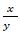class="remain-inline"> the subject.Cross multiple: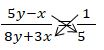5(5y – x) = 1(8y + 3X)

Expand the bracket:

25Y – 5X = 8Y + 3X

25Y- 8Y = 3X + 5X

17Y = 8X

Divide through by 8.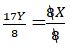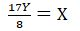Divide through by Y to make X the numerator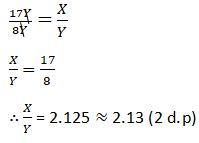2.

The angle of a sector of a circle with radius 22cm is 60o. If the sector is folded such that the straight edges coincide, forming a cone,calculate correct to one decimal place, the:

(a)

(b)

height;

(c)

volume;

of the cone;
[Take π = 227]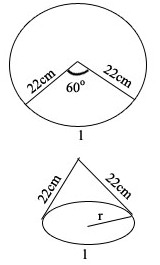From the above diagram, the length of the arc becomes the circumference of the base of the cone.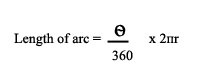radius of the circle = 22cm
θ = 60o

(a)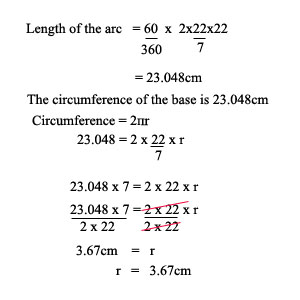⇒ The radius of the cone = 3.67cm

(b)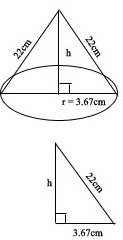The height (h) can be calculated using pythagoras theorem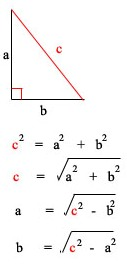The c (hypotanus is always the side facing the right angle (⊾))

⇒ 222 = 3.672 + h2
222 - 3.672 = h2
470.53 = h2
Take the square root (√) of both sides to get ride of the square
√470.53 = h
21.69 = h
⇒ The height of the cone = 21.69cm

(c)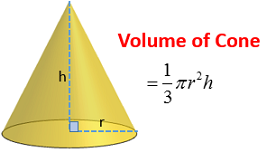Volume = ⅓ x 227x3.672 x 21.69

Volume = 306.05cm3

NB: Unit of area = unit square (e.g cm2,m2) and unit of volume = unit cubic (e.g cm3, m3)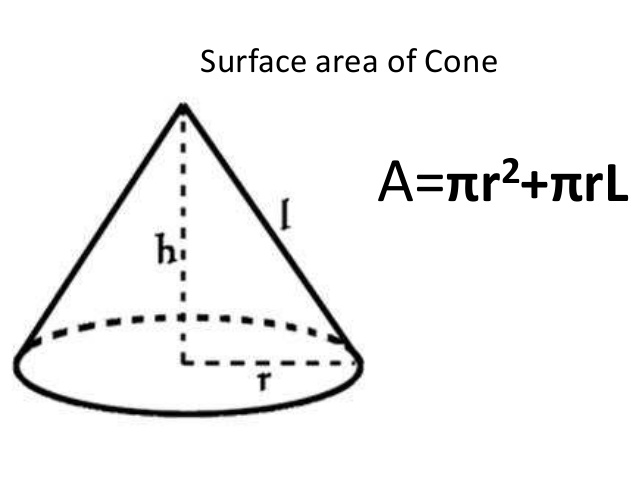3.
Without using mathematical tables or calculators, evaluate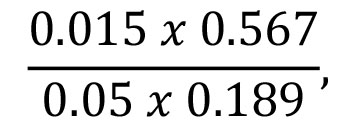leaving the answer in standard form.Run the decimal point to the right side to get whole number for easy calculation: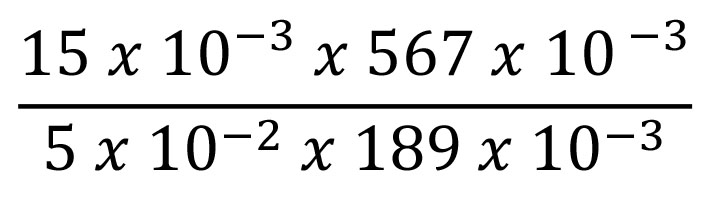Cancellation will take place here, 5 will go into 15 (3times) and 189 will go into 567 (3times). Which will give us: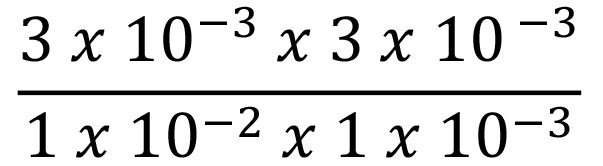Group like terms: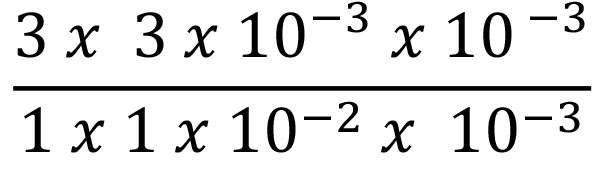Multiply like terms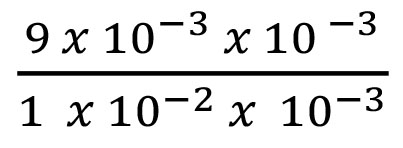Indices first law will be applied here. Which says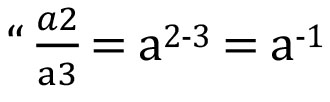“If the bases are the same take one of the base and add the exponent: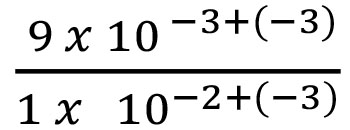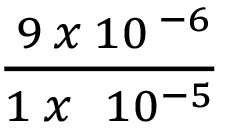Indices second law will be applied here. Which says“If the bases are the same take one of the base and subtract the exponent:

9 x 10-6– (-5)

9 x 10-14.
Factorize completely:

4b2– ab + (a + 9b)2 – a2.

4b2 – ab + (a + 9b)2 – a2

step 1: Expand (a + 9b)2 to give (a + 9b) (a + 9b)

4b2 – ab + (a + 9b) (a + 9b) – a2

step 2: Expand the bracket:

=4b2 – ab + a2 + 18ab + 81b2 – a2

step 3: Group like terms:

= 4b2 + 81b2 – ab + 18ab + a2 - a2

= 4b2 + 81b2 – ab + 18ab

= 85b2 + 17ab

step 4: Factorize 17b out:

= 17b(5b + a)

5.

The first three terms of an Arithmetic Progression (A.P:) are (x + 1),(4x - 2) and (6x - 3)

respectively.If the last term is 18, find the:

(a)

vale of x;

(b)

Sum of the terms of the progression.

Notes

Arithmetic Progression

Is a sequence of numbers such that the difference between the consecutive terms is constant.

E.g The sequence 1,3,5,7,9.. is an arithmetic progression with a common difference of 2

an = a1 + (n - 1)d

an = The value of the sequence number of on the nth position.

a1 = the first number in the sequence

d = the common difference

d = an + 1 - an (The difference between two consecutive sequence numbers)

d is the same for all consecutive numbers used for the calculator.

Thus an + 2 - an + 1 = an + 1 - an

For instance, the common difference for the sequence a1, a2, a3, a4, a5, a6 .... will be calculated as:
a2 - a1 = a3 - a2 = a5 - a4 = a6 - a5

NB: The common difference (d) is the higher sequence number value - lower previos sequence number.

For the sequence 1,3,5,7,9

⇒ d = 3 - 1 = 5 - 3 = 7 - 5 = 9 - 7 = 2

Summation of Arithmetic Progression

Sn = n/2 (a1 + an)

Sn = n/2 [2a1 + (n - 1)d]

If the last number is know the summation can also be writen as

Sn = n/2 [a1 + l]

where l = last term

(a)

A. P = (x + 1), (4x - 2) and (6x - 3)

⇒ From the knowledge of common difference calculation

(4x - 2) - (x + 1) = (6x - 3) - (4x - 2)

NB Common difference is the same for all consecutive terms

⇒ 4x - 2 - x - 1 = 6x - 3 - 4x - -2

4x - 2 - x - 1 = 6x - 3 - 4x + 2

3x - 3 = 2x - 1

3x - 2x = -1 + 3

x = 2

(b)

Since we are given the last term, we are to use the summation formula with the last term in it.

Sn = n2 [a1 + l]

We need to calculate the (n) position for the last number (l)

an = a1 + (n - 1)d

x = 2

⇒ first term = x + 1 = 2 + 1 = 3

Second term = 4x - 2 = 4 x 2 - 2 = 6

Third term = 6 x 2 - 3 = 9

The sequence = 3,6,9...18

⇒ a1 = 3

d = 6 - 3 = 9 - 6 = 3

But an = a1+(n-1)d
an = 18
a1 = 3
d = 3

⇒ 18 = 3+(n-1)x3
18 = 3+3n-3
18 = 3n
3n3 = 183
n = 6

⇒ Sn = n2[a1+l]
Sn = 62[3+18]
Sn = 63

6.
Z varies directly as x and inversely as twice the cube root of y. If Z = 8, when x = 4 and y = 1/8 find the relation for y in terms of x and Z.

Z varies directly as x and inversely as twice the cube root of y is written as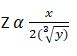which is equal to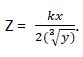k is constant

Since Z = 8, x = 4, y = 1/8, substitute them in place of the variables.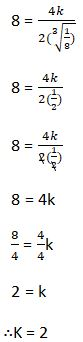This gives: